• Products
• Company
• Resources
• Docs
• Pricing

# How To Train a Fake News Detector Using ECTF Dataset?

How to train a fake news detector using ECTF dataset after extracting new fields by Twitter API? How can new fields show us further insights?In recent years, social media sites have become widespread, and the number of people using them has been large and it continues to grow. People now prefer news pages on social media sites to follow the news, and those pages have become the only news source for many people. As a result, it has become easy to spread fake news and rumors and maliciously mislead people. To avoid those dangers, systems that automatically detect fake news are needed.

## Goals

In this tutorial, we will download the ECTF dataset, which is published by Delhi Technological University. After that, we will extract more data about the tweets using Twitter API. Subsequently, we will use the enriched dataset for various visualizations to get further insights into the data. Finally, we will train 2 different supervised learning models to distinguish fake news automatically. One is machine learning-based and the other is deep learning-based.

## ECTF Dataset

ECTF is a dataset for Twitter fake news detection in the Covid-19 domain. It consists of 3 files; fake.csv which contains fake tweets, genuine.csv which contains real tweets, and unlabelled.csv which contains mixed unlabeled data. In this tutorial, we will use both genuine.csv and fake.csv. Each file of them contains 2,000 tweets with the following fields:

1. Index: file-specific field

2. Tweet ID: Each object within Twitter - a Tweet, Direct Message, User, List, and so on - has a unique ID. Twitter IDs are unique 64-bit unsigned integers, which are based on time. We will use this field to retrieve more data about the tweets.

3. The text of the tweet

The Twitter API is a set of programmatic endpoints that can be used to access the data on Twitter programmatically, which is precious for data-centric research. This API allows us to find and retrieve different resources on Twitter, like tweets, users, trends,.... etc. On the other hand, we have Tweepy, which is a Python library for accessing the Twitter API.

Log in to your Twitter account and go to the developer portal. Then, create a new project, and go to the dashboard. Click the buttons shown in the following image to generate the tokens and save them in a safe place: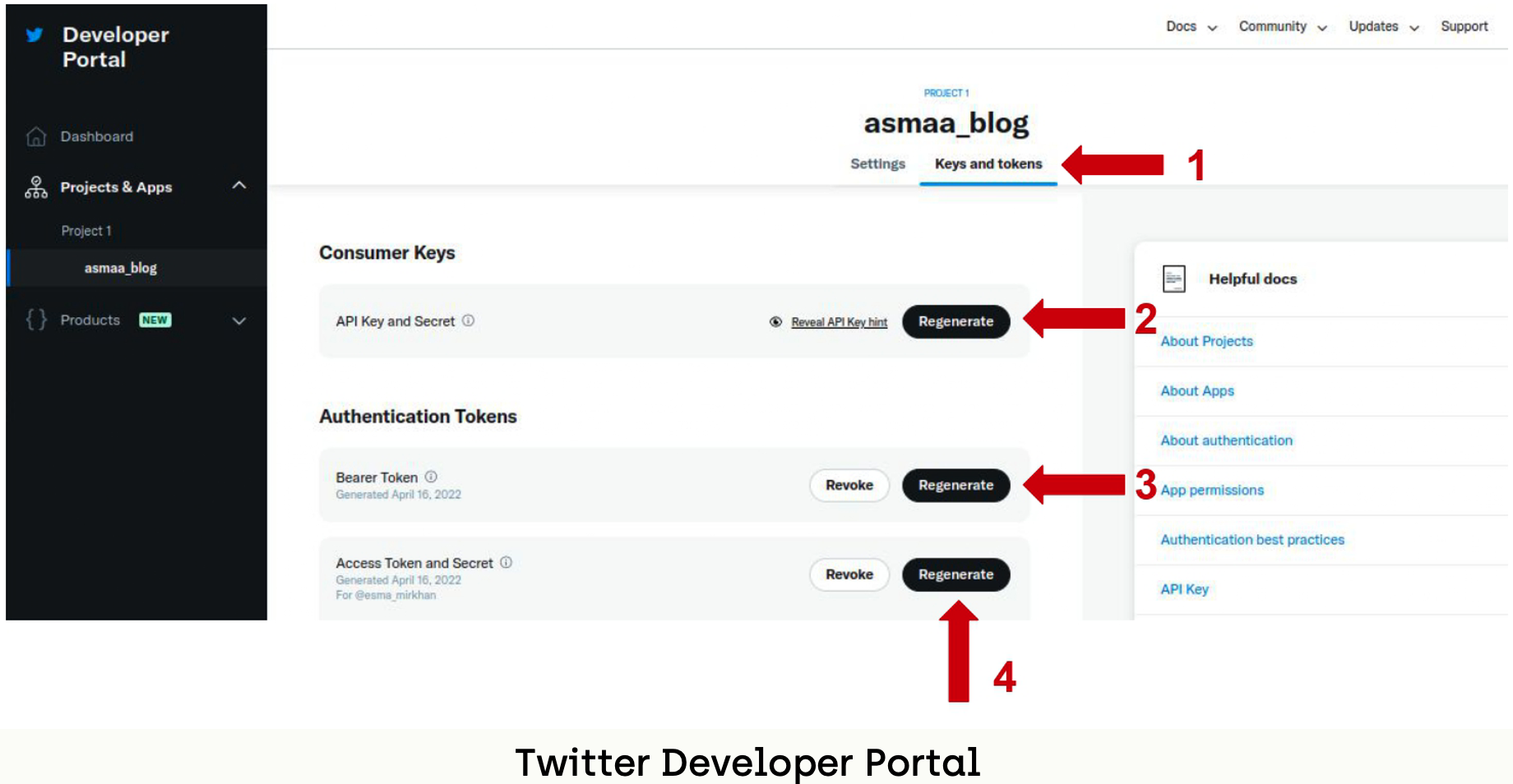## Environment Setup

### Environment Info

All the codes in this tutorial are tested against the following versions of packages:

Module/PackageVersion
Numpy1.19.1
NLTK3.5
Pandas1.1.3
Python3.7
Scikit-Learn0.24.2
SciPy1.6.2
Seaborn0.11.0
Tensor Flow2.4.1
TextBlob0.15.3
Tweepy4.8.0

First of all, let us start by creating a new Conda environment named fnd and activating it:

``````conda create -n fnd python=3.7
conda activate fnd``````

Now, we can install the required packages for the first part of the tutorial:

``````# in fnd environment
conda install -c conda-forge tweepy
conda install -c anaconda pandas
``````

### Step 1 - Exploring the Data

Firstly, let us download the dataset from ECTF Dataset GitHub Repository and then convert them to Pandas data frame using the following code snippet:

``````import pandas as pd

Now, the tweets are saved in real_data and fake_data like the following: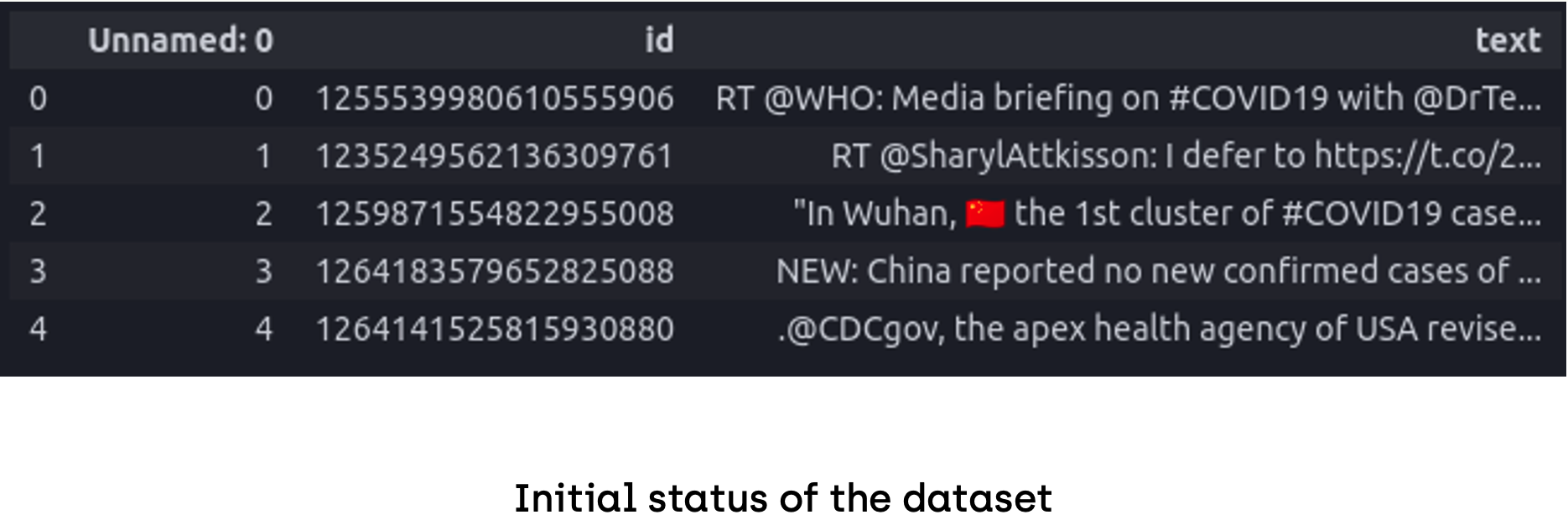### Step 2 - Twitter API Access

To be able to use Twitter API we have to initialize a Client object using the tokens we got in the previous section:

``````import tweepy
import requests

CONSUMER_API_KEY = "PUT_YOUR_CONSUMER_API_KEY"
CONSUMER_API_KEY_SECRET = "PUT_YOUR_CONSUMER_API_KEY_SECRET"

ACCESS_TOKEN = "PUT_YOUR_ACCESS_TOKEN"
ACCESS_TOKEN_SECRET = "PUT_YOUR_ACCESS_TOKEN_SECRET"

BEARER = "PUT_YOUR_BEARER"

client = tweepy.Client(bearer_token=BEARER, consumer_key=CONSUMER_API_KEY,
consumer_secret=CONSUMER_API_KEY_SECRET,
access_token=ACCESS_TOKEN,
access_token_secret=ACCESS_TOKEN_SECRET,
return_type=requests.Response
)``````

Now, since we have access to resources on Twitter, we can start fetching the data for our analysis. To get more information about the tweets depending on tweet_id we can use the get_tweets() function. Let's start by defining some constants;

``````batch_size = 100
tweet_fields = ["public_metrics", "created_at", "entities", "author_id"]
user_fields = ["name", "username", "location", "public_metrics"]``````
•  batch_size: Defines the number of tweets to be fetched per request, referring to the documentation of get_tweets(); up to 100 are allowed in a single request.

•  tweet_fields: Defines the attributes that we want to get about the tweets. All attributes are listed in the documentation of get_tweets().

• user_fields: Defines the attributes that we want to get about the owner of the tweets.

To fetch the attributes for the whole dataset we have to split it into batches with a batch size of 100 and request the data batch by batch. We can achieve that using the following functions:

• Function to divide the dataset into batches

``````def batch(iterable, n=1):
l = len(iterable)
for ndx in range(0, l, n):
yield iterable[ndx:min(ndx + n, l)]
-	Function to retrieve data using get_tweets() function:
def fetch_tweet_fields(dataset):
all_tweets = dict((k, []) for k in ["data", "users", "errors"])

ids = list(dataset["id"])
for id_batch in batch(ids, batch_size):
tweet_batch = client.get_tweets(id_batch,
tweet_fields= tweet_fields,
user_fields=user_fields,
expansions="author_id",
user_auth=True
)

if tweet_batch.json().get("data"): all_tweets["data"].extend(tweet_batch.json()["data"])
if tweet_batch.json().get("includes"): all_tweets["users"].extend(tweet_batch.json()["includes"]["users"])
if tweet_batch.json().get("errors"): all_tweets["errors"].extend(tweet_batch.json()["errors"])

return all_tweets``````

We can call the functions in the following way:

``````real_tweets = fetch_tweet_fields(real_data)

fake_tweets = fetch_tweet_fields(fake_data)``````
##### New Fields Description

Now, both real_tweets and fake_tweets are dicts that have 3 keys; "data", "users" and "errors".

- data: list of json objects that contain details about the tweets

- users: list of json objects that contain details about the authors

- errors: list of json objects that contain details about the error messages

Note: The errors field shows us that some of the tweets have been deleted or made private. In this situation, we can only retrieve data from 1913 tweets from the real ones and 1030 tweets from the fake ones. This can be an important meaningful indication that lots of fake news are deleted.

### Step 4 - Converting to DataFrame

For easier operations, we can convert the dictionaries to pandas data frames in the following way:

``````real_tweets_data = pd.json_normalize(real_tweets['data'])
real_tweets_users = pd.json_normalize(real_tweets['users'])

fake_tweets_data = pd.json_normalize(fake_tweets['data'])
fake_tweets_users = pd.json_normalize(fake_tweets['users'])``````

### Renaming ID Fields

To avoid confusion, we will rename the id field in data data frame to tweet_id and the id field of users data frame to author_id:

``````real_tweets_data.rename(columns={"id":"tweet_id"}, inplace=True)
fake_tweets_data.rename(columns={"id":"tweet_id"}, inplace=True)

real_tweets_users.rename(columns={"id":"author_id"}, inplace=True)
fake_tweets_users.rename(columns={"id":"author_id"}, inplace=True)``````

### Drop Duplicates

Since we have repeated user names, we can remove duplicated ones using the drop_duplicates() function:

``````real_tweets_users =
real_tweets_users.loc[real_tweets_users.astype(str).drop_duplicates(subset='author_id', keep="last").index]
fake_tweets_users =
fake_tweets_users.loc[fake_tweets_users.astype(str).drop_duplicates(subset='author_id', keep="last").index]``````

### Merging Dataframes

For easier processing, we can merge data and users' data frames on the author_id field.

``````merged_real_tweets=pd.merge(real_tweets_data, real_tweets_users, on='author_id')
merged_fake_tweets=pd.merge(fake_tweets_data, fake_tweets_users, on='author_id')``````

### Beautifying Field Names

Since we got the data from Twitter API, some of the names are long and not practical to use, we can beautify names using the following script:

``````def beautify_field_names(data):
data = data.rename(columns={"entities.hashtags":"hashtags",
"entities.mentions":"mentions",
"entities.urls":"urls",
"public_metrics.retweet_count": "retweet_count",
"public_metrics.like_count": "like_count",
"public_metrics.quote_count": "quote_count",
"entities.annotations": "annotations",
"name":"retweeter_name",
"public_metrics.followers_count":"retweeter_followers_count",
"public_metrics.following_count":"retweeter_following_count",
"public_metrics.tweet_count":"retweeter_tweet_count",
"public_metrics.listed_count":"retweeter_listed_count"}, inplace=True)

beautify_field_names(merged_real_tweets)
beautify_field_names(merged_fake_tweets)``````

### Beautifying Fields Contents

Since we got the data from Twitter API, fields that contain lists are downloaded as JSON with a lot of details that are not important in the context of this tutorial. Also, some of the fields are not available for all records. For example, location info is available only for some of the tweets and the others are None. It is better to replace Nones with empty strings to avoid string operation errors. So, we can convert lists of json objects to lists and replace Nones with empty strings:

``````def beautify_items_with_lists(data):
data["location"] = data["location"].fillna("")
data["hashtags"] = data["hashtags"].fillna("").apply(list)
data["mentions"] = data["mentions"].fillna("").apply(list)
data["urls"] = data["urls"].fillna("").apply(list)
data["annotations"] = data["annotations"].fillna("").apply(list)
data["hashtags"] = data["hashtags"].apply(lambda x: [item["tag"] for item in x])
data["mentions"] = data["mentions"].apply(lambda x: [item["username"] for item in x])
data["urls"] = data["urls"].apply(lambda x: [item["url"] for item in x])

beautify_items_with_lists(merged_real_tweets)
beautify_items_with_lists(merged_fake_tweets)``````

A lot of tweets contain links in their texts which can affect the text-based analysis that we are going to do. Since we have URLs in a separate column, we can remove them from the texts without data loss.

``````merged_real_tweets["text"] = merged_real_tweets['text'].replace(r'http\S+', '', regex=True).replace(r'www\S+', '', regex=True)
merged_fake_tweets["text"] = merged_fake_tweets['text'].replace(r'http\S+', '', regex=True).replace(r'www\S+', '', regex=True)``````

### Step 6 - Retrieving Owner User Name

A big part of the dataset contains retweeted tweets and it will be more meaningful to analyze the original tweeters instead of the retweeters. Since the retweeted tweets start with RT @username, we can retrieve the original user name depending on the tweet text without using Twitter API:

``````def get_owner_username(tweet):
text = tweet["text"]
if text.startswith("RT @"):
return text[text.index("@")+1:text.index(":")]
else:

### Step 7 - Feature Selection

Since we have too many fields in the data frame, we will select a subset of features to analyze in this tutorial.

``````sub_set = ['text', 'mentions', 'urls', 'hashtags', 'retweet_count', 'reply_count', 'like_count',
'retweeter_following_count', 'retweeter_tweet_count']
sub_real_tweets = merged_real_tweets[sub_set]
sub_fake_tweets = merged_fake_tweets[sub_set]``````

### Subjectivity & Polarity Scores

TextBlob is a Python library for processing textual data. It provides a simple API for diving into common natural language processing (NLP) tasks such as part-of-speech tagging, noun phrase extraction, sentiment analysis, classification, translation, and more.

We will use this package to extract semantic features from the dataset, like objectivity and polarity scores depending on the tweets' texts. Let's start by installing the textblob conda package.

``````# in fnd environment
conda install -c conda-forge textblob -y``````

To get subjectivity scores, we can use sentiment.subjectivity attribute, which returns afloat within the range [0.0, 1.0] where 0.0 is very objective, and 1.0 is very subjective.

``````from textblob import TextBlob

def getSubjectivity(text):
return TextBlob(text).sentiment.subjectivity``````

In a similar way, we can extract polarity scores by using sentiment.polarity attribute, which returns afloat within the range [-1.0, 1.0] where -1 defines a negative sentiment and 1 defines a positive sentiment.

``````def getPolarity(text):
return TextBlob(text).sentiment.polarity``````

Now, we can calculate subjectivity and polarity scores and add them to the data frames:

``````real_polarities = [getPolarity(content["text"]) for index, content in sub_real_tweets.iterrows()]
real_subjectivities = [getSubjectivity(content["text"]) for index, content in sub_real_tweets.iterrows()]
sub_real_tweets["polarity"] = real_polarities
sub_real_tweets["subjectivity"] = real_subjectivities

fake_polarities = [getPolarity(content["text"]) for index, content in sub_fake_tweets.iterrows()]
fake_subjectivities = [getSubjectivity(content["text"]) for index, content in sub_fake_tweets.iterrows()]
sub_fake_tweets["polarity"] = fake_polarities
sub_fake_tweets["subjectivity"] = fake_subjectivities``````

### Plotting Results

Let's start plotting by defining labels:

``````polarity_labels = ["Positive", "Negative", "Neutral"]
subjectivity_labels = ["Subjective", "Objective", "Neutral"]``````

Subsequently, we can label records depending on polarity and subjectivity scores:

``````pos_size_real = sub_real_tweets.loc[sub_real_tweets['polarity']>0].shape
neg_size_real = sub_real_tweets.loc[sub_real_tweets['polarity']<0].shape
neu_size_real = sub_real_tweets.loc[sub_real_tweets['polarity']==0].shape
sizes_real = [pos_size_real, neg_size_real, neu_size_real]

pos_size_fake = sub_fake_tweets.loc[sub_fake_tweets['polarity']>0].shape
neg_size_fake = sub_fake_tweets.loc[sub_fake_tweets['polarity']<0].shape
neu_size_fake = sub_fake_tweets.loc[sub_fake_tweets['polarity']==0].shape
sizes_fake = [pos_size_fake, neg_size_fake, neu_size_fake]``````

Now, we can plot the number of records per class as a pie chart:

``````import matplotlib.pyplot as plt
fig1, ax1 = plt.subplots(nrows=1, ncols=2, figsize=(12,7))

ax1.pie(sizes_real,  labels=polarity_labels, autopct='%1.1f%%',

ax1.axis('equal')
ax1.title.set_text("Real News")

ax1.pie(sizes_fake,  labels=polarity_labels, autopct='%1.1f%%',
ax1.axis('equal')
ax1.title.set_text("Fake News")

plt.show()``````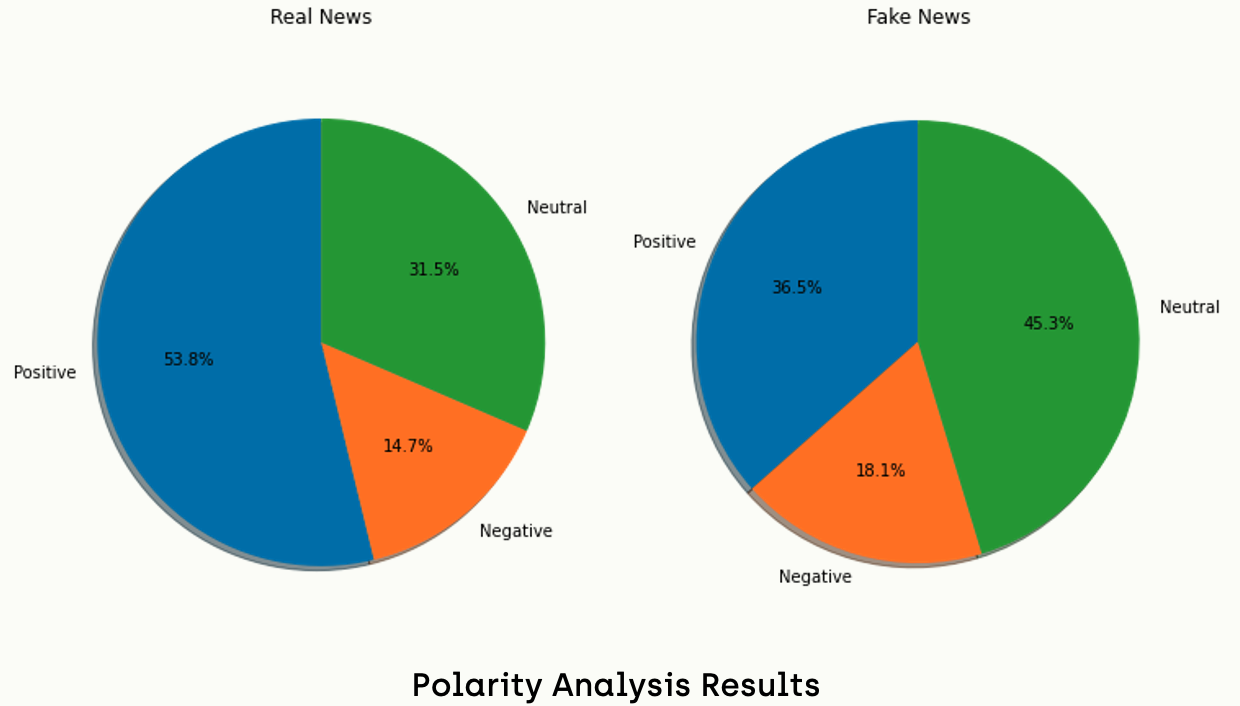Obviously, we can say that real news tends to be more positive than fake news. On the other hand, fake news tends to be more neutral than fake news.

If we do the same analysis on the subjectivity field, we get the following results: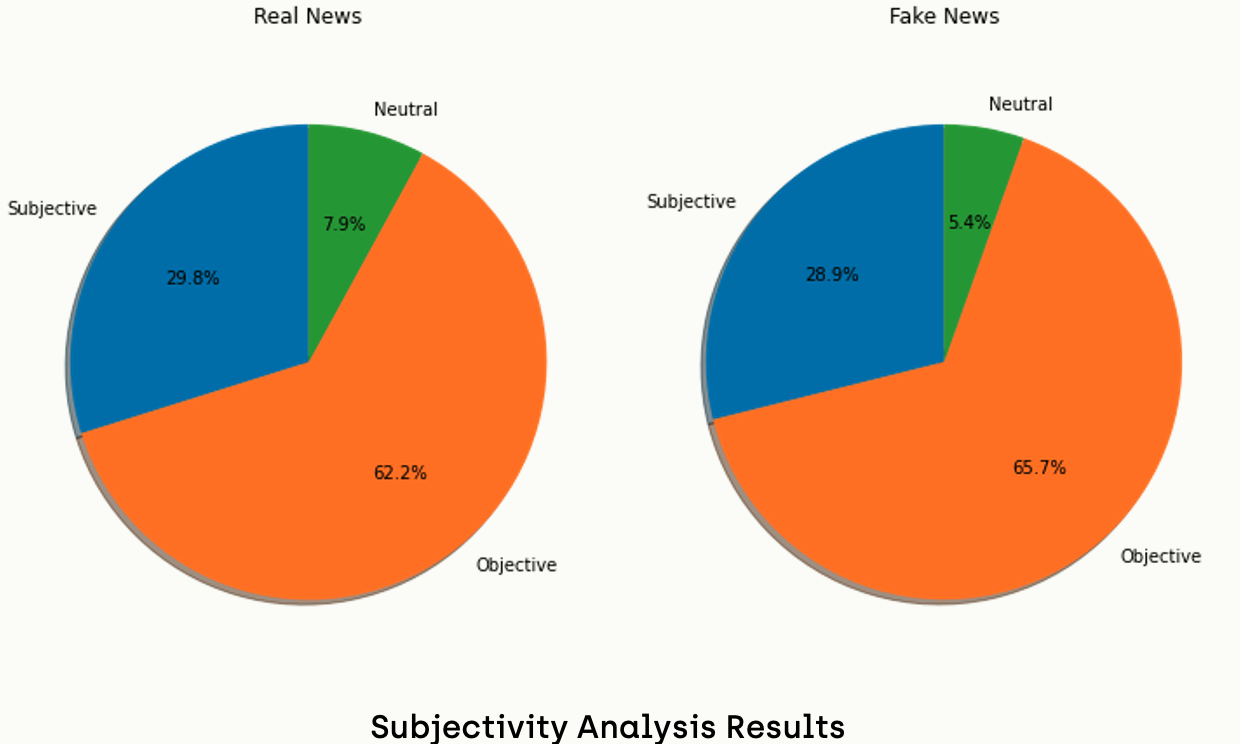Conversely, we cannot draw similar conclusions from subjectivity analysis since the results are very close.Discover how training data can make or break your AI projects, and how to implement the Data Centric AI philosophy in your ML projects.

### Counting Words and Pairs

In this section, we will focus on the repetition of words and phrases in the tweets and then we will interpret the results after plotting them as bar charts. Let's start by installing the NLTK package, which stands for Natural Language Toolkit. It is a platform used for building Python programs that work with human language data for application in statistical natural language processing.

``````# in fnd environment
conda install -c anaconda nltk``````

To get the most repeated words, we have to apply some transformations to the tweets' texts. For example, we will apply lemmatization, which is a linguistic term that means grouping together words with the same root. Also, we will convert all letters in tweets to lowercase because "Covid" and "covid" must be considered the same word in terms of word counting. In addition, we can remove stop words because they are not useful in this type of analysis.

We can use the following function to get the most repeated words and pairs in our dataset:

``````from nltk.tokenize import word_tokenize
from nltk.corpus import stopwords
from nltk.stem import WordNetLemmatizer
from nltk import ngrams
from collections import Counter

def word_frequency(sentences):
all_sentences = " ".join(sentences)

tokens = word_tokenize(all_sentences)
tokens = [t.lower() for t in tokens]
stopwords_extended = stopwords.words('english')
stopwords_extended.extend(["amp", "rt"])
tokens = [t for t in tokens if t not in stopwords_extended]
tokens = [t for t in tokens if t.isalpha()]
lemmatizer = WordNetLemmatizer()
tokens = [lemmatizer.lemmatize(t) for t in tokens]

counted_words = Counter(tokens)
counted_pairs = Counter(ngrams(tokens, 2))

word_freq = pd.DataFrame(counted_words.items(), columns=[
'word', 'frequency']).sort_values(by='frequency', ascending=False)

word_pairs = pd.DataFrame(counted_pairs.items(), columns=[
'pairs', 'frequency']).sort_values(by='frequency', ascending=False)

return word_freq, word_pairs

word_freq_real, word_pairs_real = word_frequency(sub_real_tweets["text"])
word_freq_fake, word_pairs_fake = word_frequency(sub_fake_tweets["text"])``````

### Plotting Bar Charts

We can use the barplot function from the seaborn package to plot the results as a bar chart.

To install the seaborn package:

``````# in fnd environment
conda install -c anaconda seaborn``````

To plot the results:

``````import seaborn as sns
fig, axes = plt.subplots(2, 1, figsize=(12, 30))
sns.barplot(ax=axes, x='frequency', y='pairs',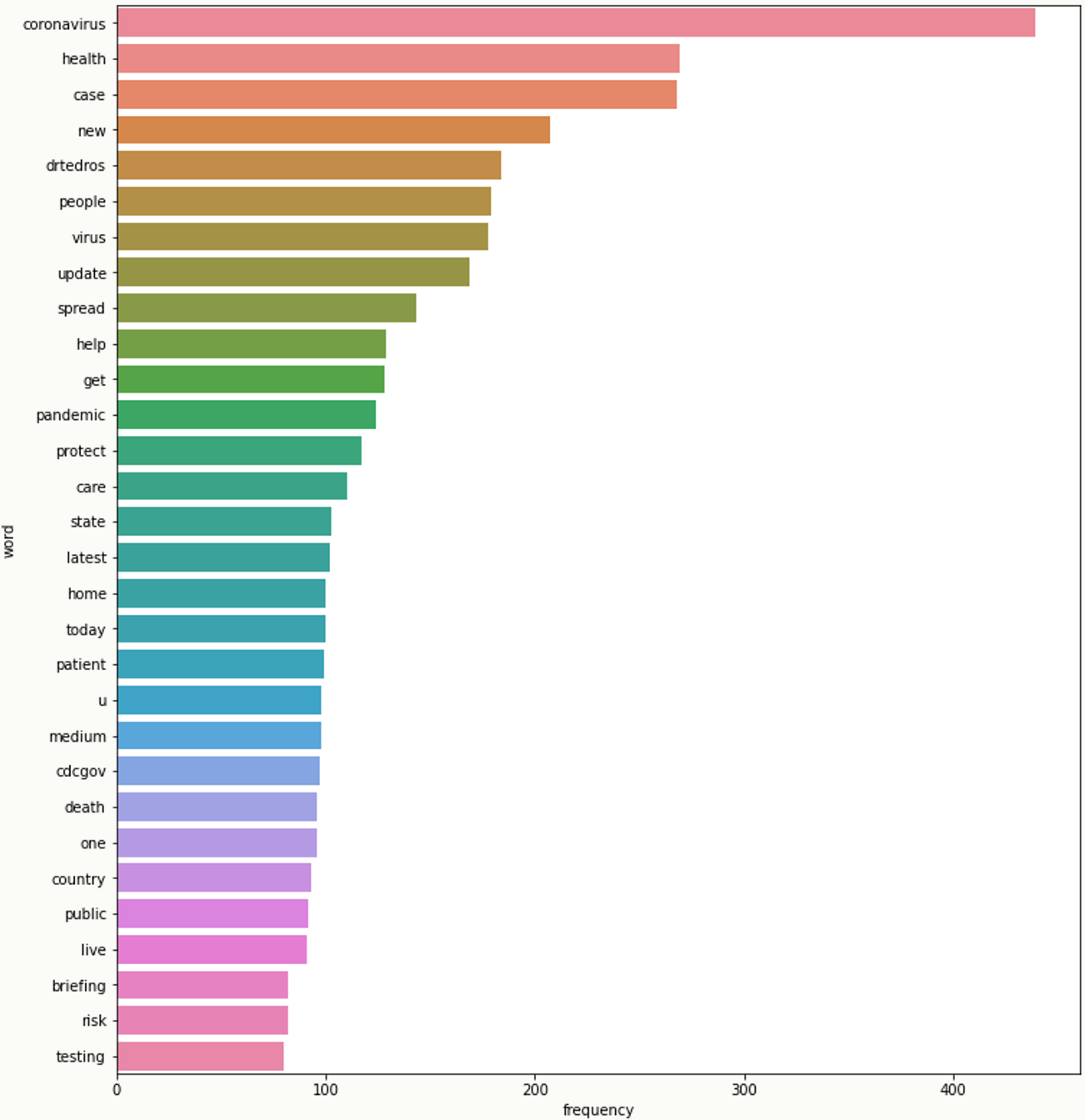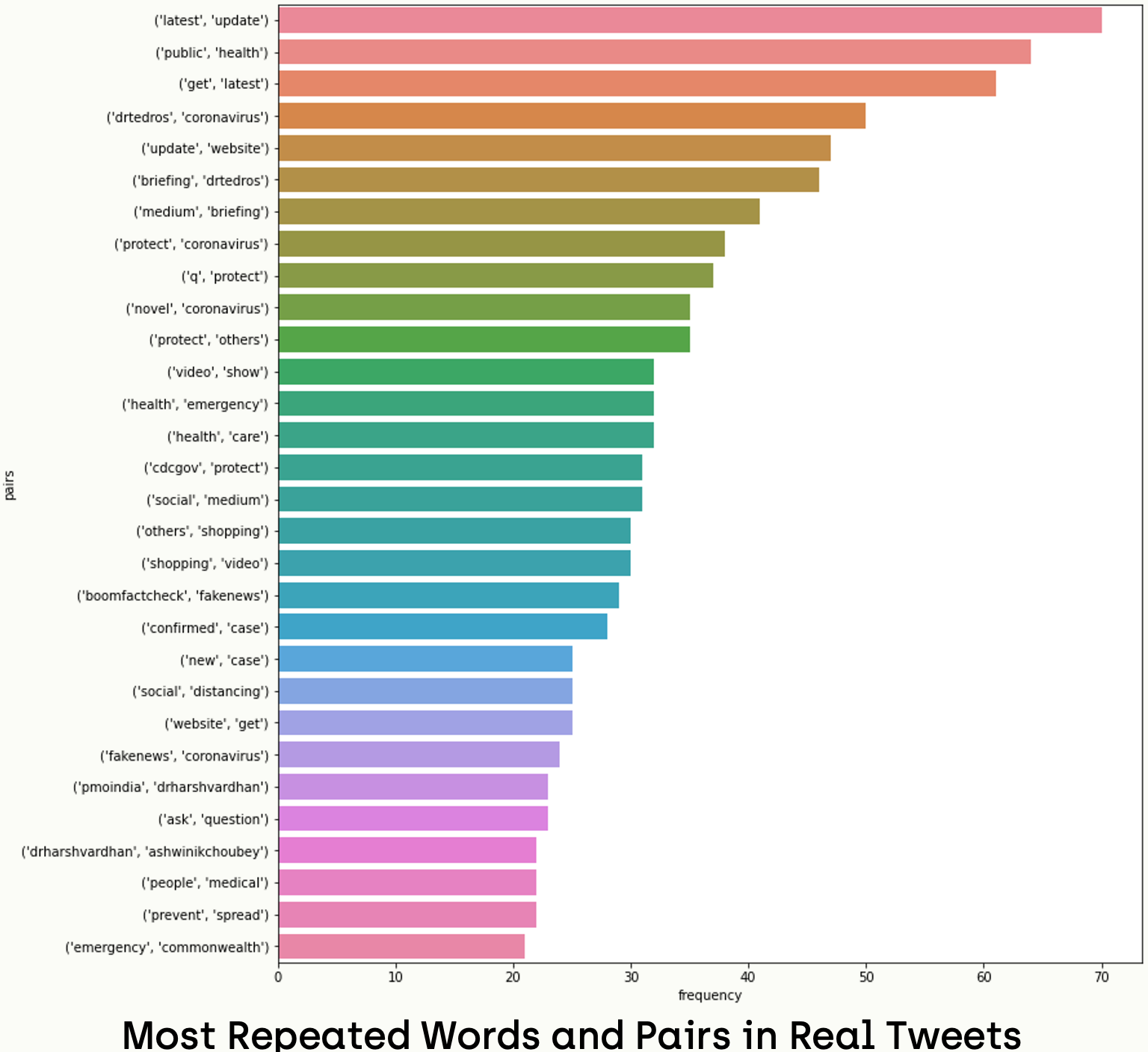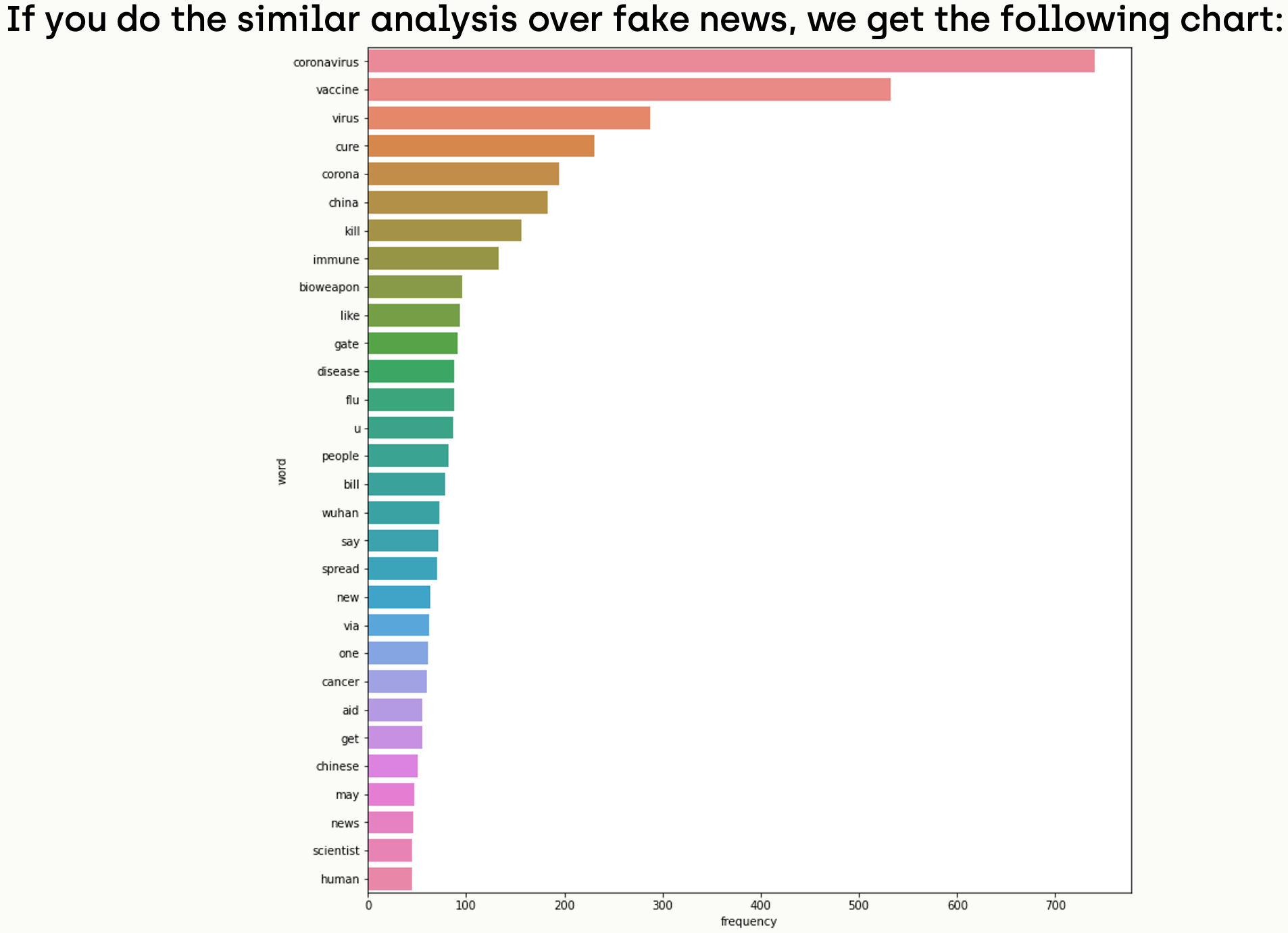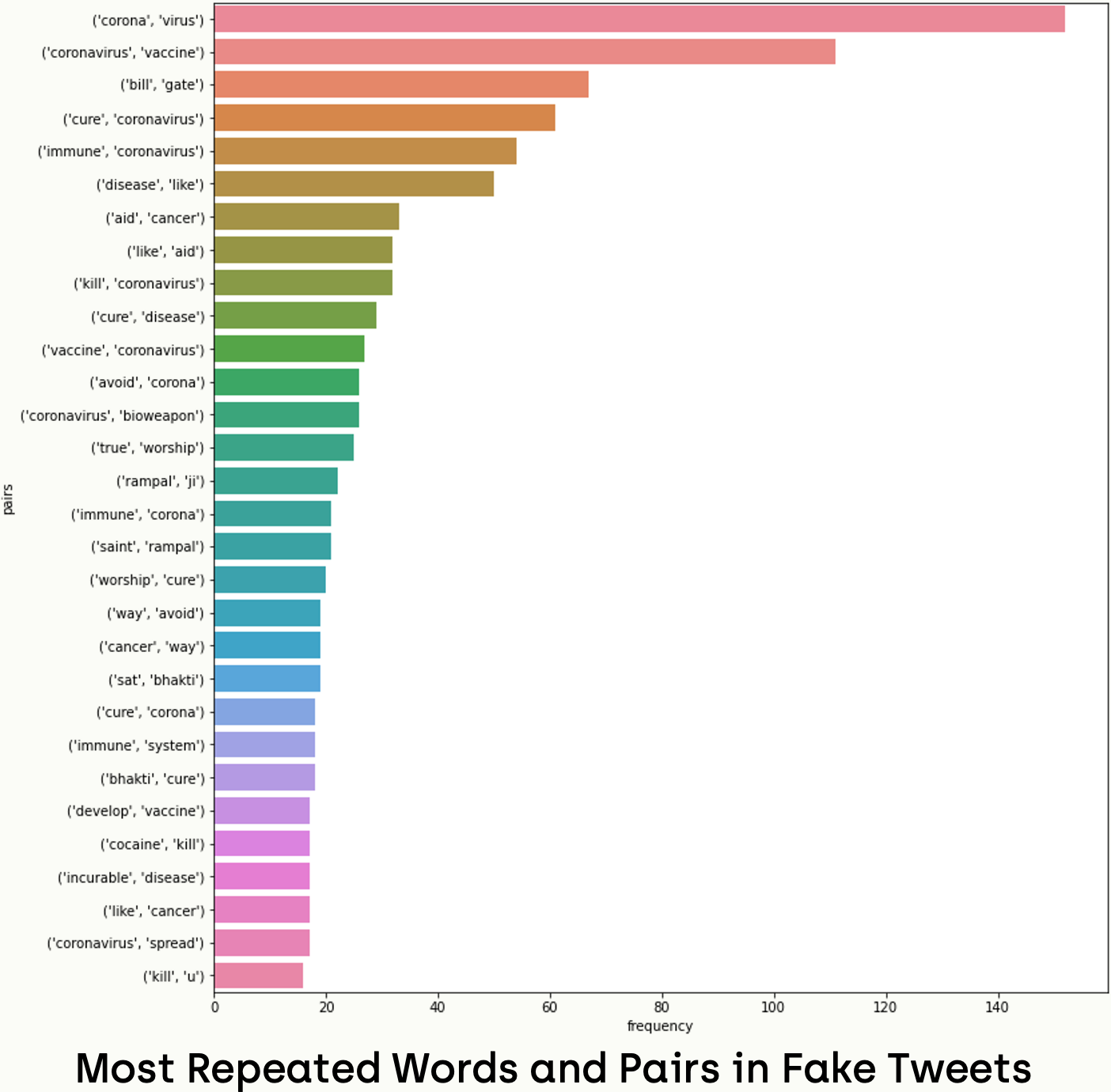Accordingly, we notice that writers of real news used more scientific and unbiased words like ("health", "case", "help", "latest update", "public health", "protect others"). While tweets of fake news tended to have biased and slang words like ("kill", "gates", "bioweapon", "china", "bill gates", "coronavirus bioweapon").

### WordCloud Visualization

Another way for visualizing repeated words is WordCloud, which shows the most frequent words with bigger and bolder letters and with different colors. We can implement that in Python using the word cloud package. Let's start by installing the package:

``````# in fnd environment
conda install -c conda-forge wordcloud``````

We can create the word cloud by converting words data frames to Python dictionaries:

``````from wordcloud import WordCloud
real_pair_dict = dict()
for index, content in word_freq_real.iterrows():
real_pair_dict[content["word"]] = content["frequency"]

fake_pair_dict = dict()
for index, content in word_freq_fake.iterrows():
fake_pair_dict[content["word"]] = content["frequency"]
real_wordcloud = WordCloud(collocations=False).generate_from_frequencies(real_pair_dict)
fake_wordcloud = WordCloud(collocations=False).generate_from_frequencies(fake_pair_dict)``````

After that, we can visualize them using the matplotlib library:

``````fig2, ax3 = plt.subplots(nrows=1, ncols=2, figsize=(20,15))

ax3.imshow(real_wordcloud, interpolation='bilinear')
ax3.title.set_text("Real News")
ax3.axis("off")
ax3.imshow(fake_wordcloud, interpolation='bilinear')
ax3.title.set_text("Fake News")
ax3.axis("off")

plt.axis("off")
plt.show()``````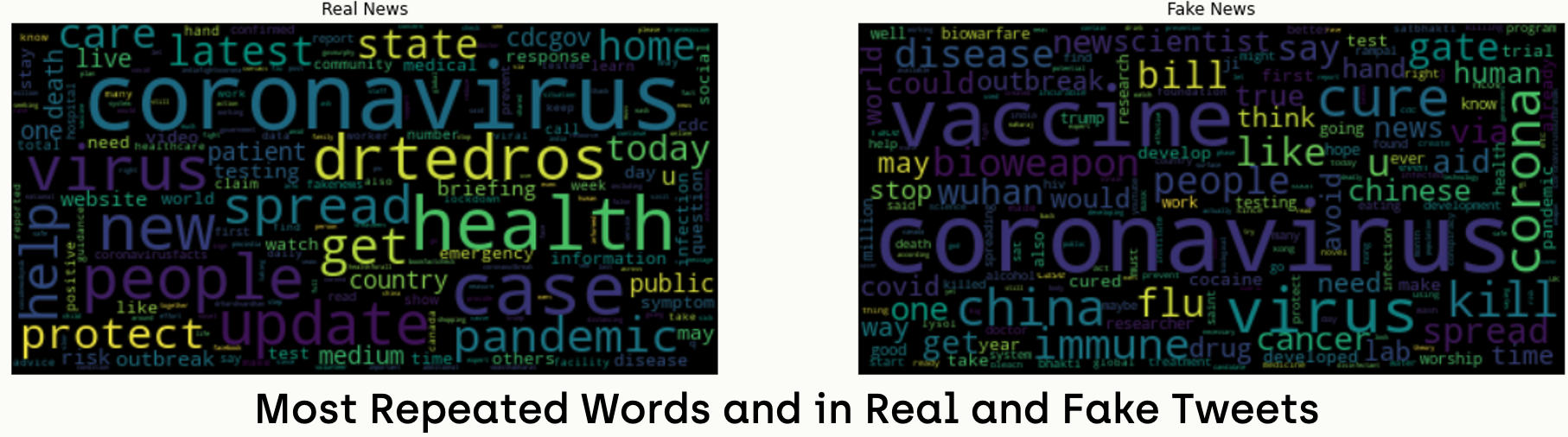We can do similar operations over the pairs data frame and get the following result: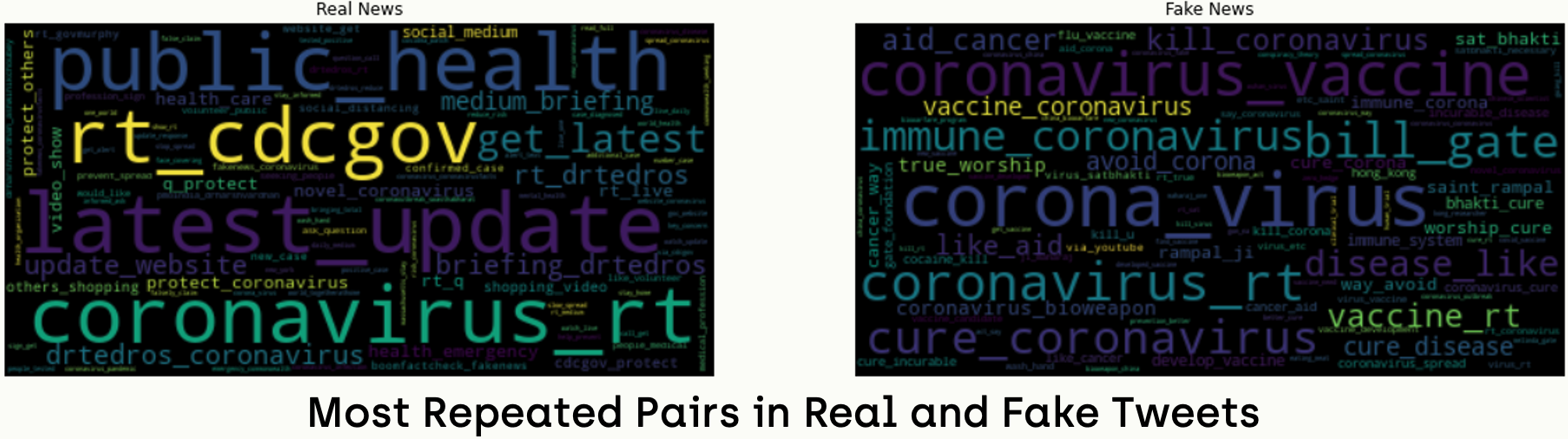### Step 10 - Hashtag and Mention Analysis

In this section, we will write a function that counts the repeated values in hashtags and mentions fields. Also, for this task, we will replace all letters with lowercase for meaningful analysis.

``````def field_frequency(values):
joined_values = " ".join(values)

tokens = word_tokenize(joined_values)
tokens = [t.lower() for t in tokens]

counted = Counter(tokens)

word_freq = pd.DataFrame(counted.items(), columns=[
'field', 'frequency']).sort_values(by='frequency', ascending=False)
return word_freq``````

### Hashtag Analysis

Since our function is ready, we can start analyzing the data. Firstly, we will retrieve all hashtags in the dataset and pass them to the counting function; after that, we will plot the most repeated hashtags.

``````all_real_tags = []
[all_real_tags.extend(tags) for tags in sub_real_tweets['hashtags']]
tag_freq_real = field_frequency(all_real_tags)
fig, axes = plt.subplots(1, 1, figsize=(12, 10))
sns.barplot(ax=axes, x='frequency', y='field',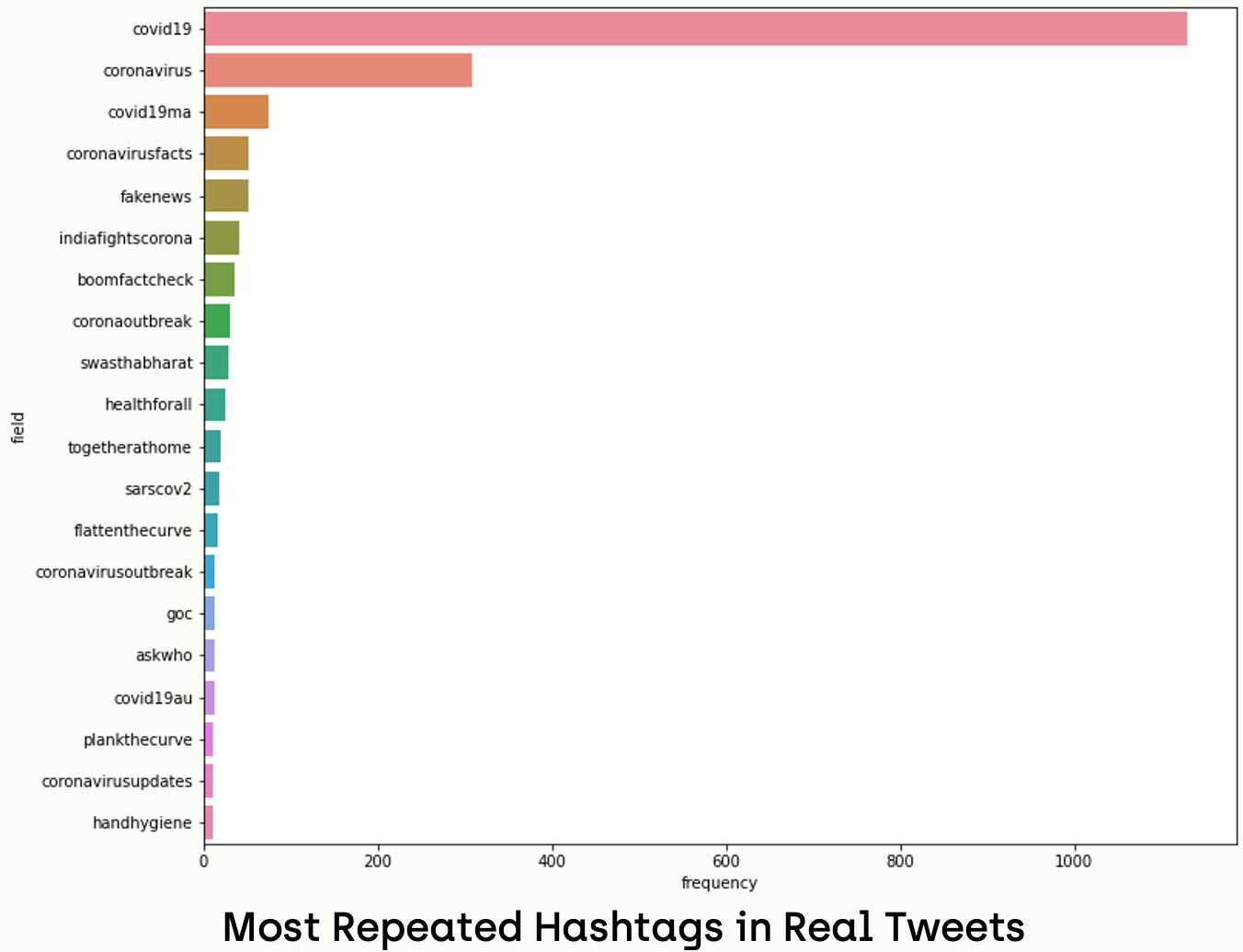Executing a similar script over fake data leads to the following chart: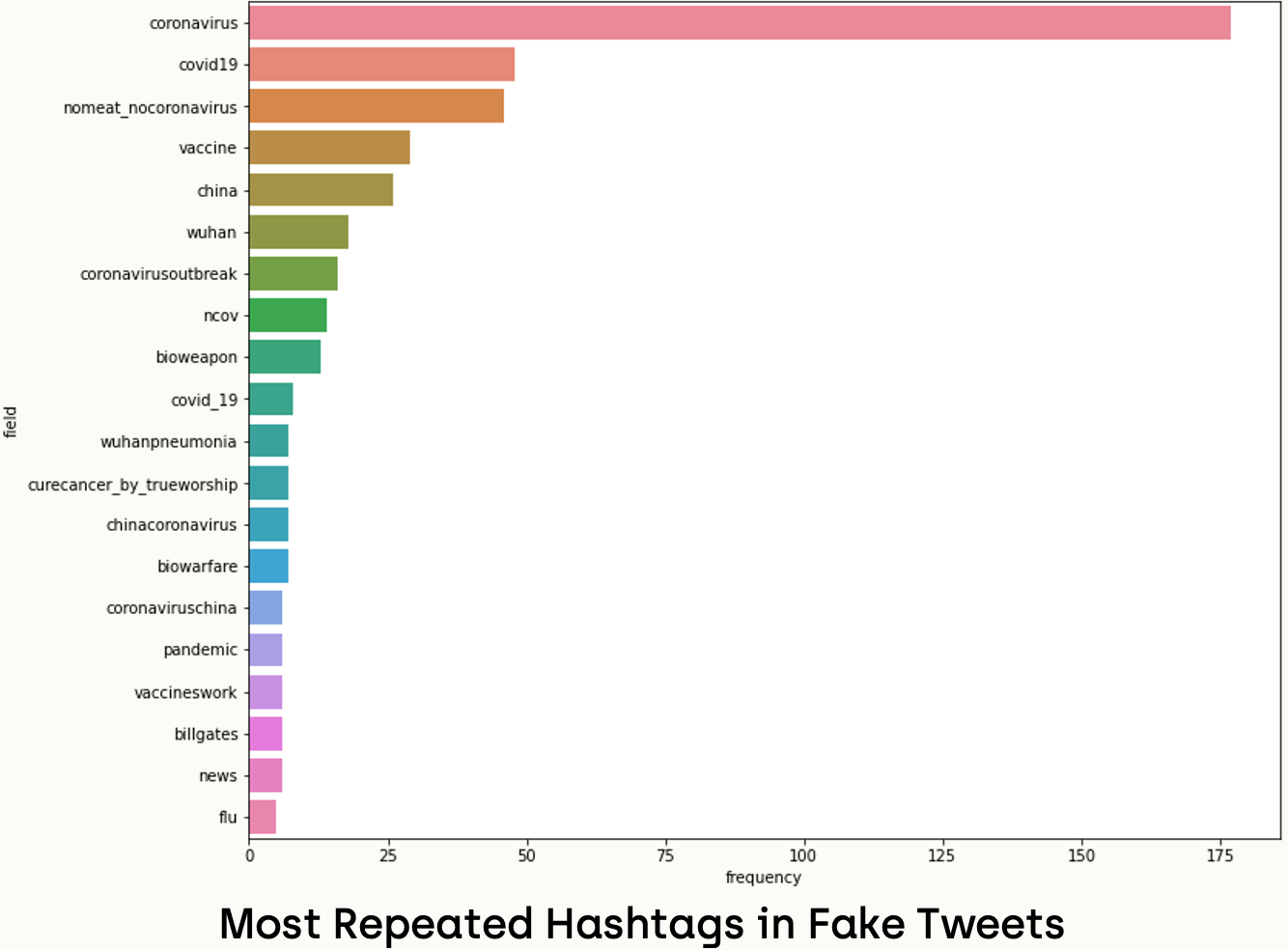The hashtags in real news like ("CoronaVirusFacts", "HealthForAll", "CoronavirusUpdates", and "TogetherAtHome") were more scientific and unbiased. In contrast with the fake news, they were biased and slang, like ("BillGates", "NoMeat_NoCoronaVirus", "Wuhan", "ChinaCoronavirus", and "China").

### Mention Analysis

In a similar way, we can apply the mentioned analysis too.

``````all_real_mentions = []
[all_real_mentions.extend(tags) for tags in sub_real_tweets['mentions']]
tag_freq_real = field_frequency(all_real_mentions)
fig, axes = plt.subplots(1, 1, figsize=(12, 10))
sns.barplot(ax=axes, x='frequency', y='field',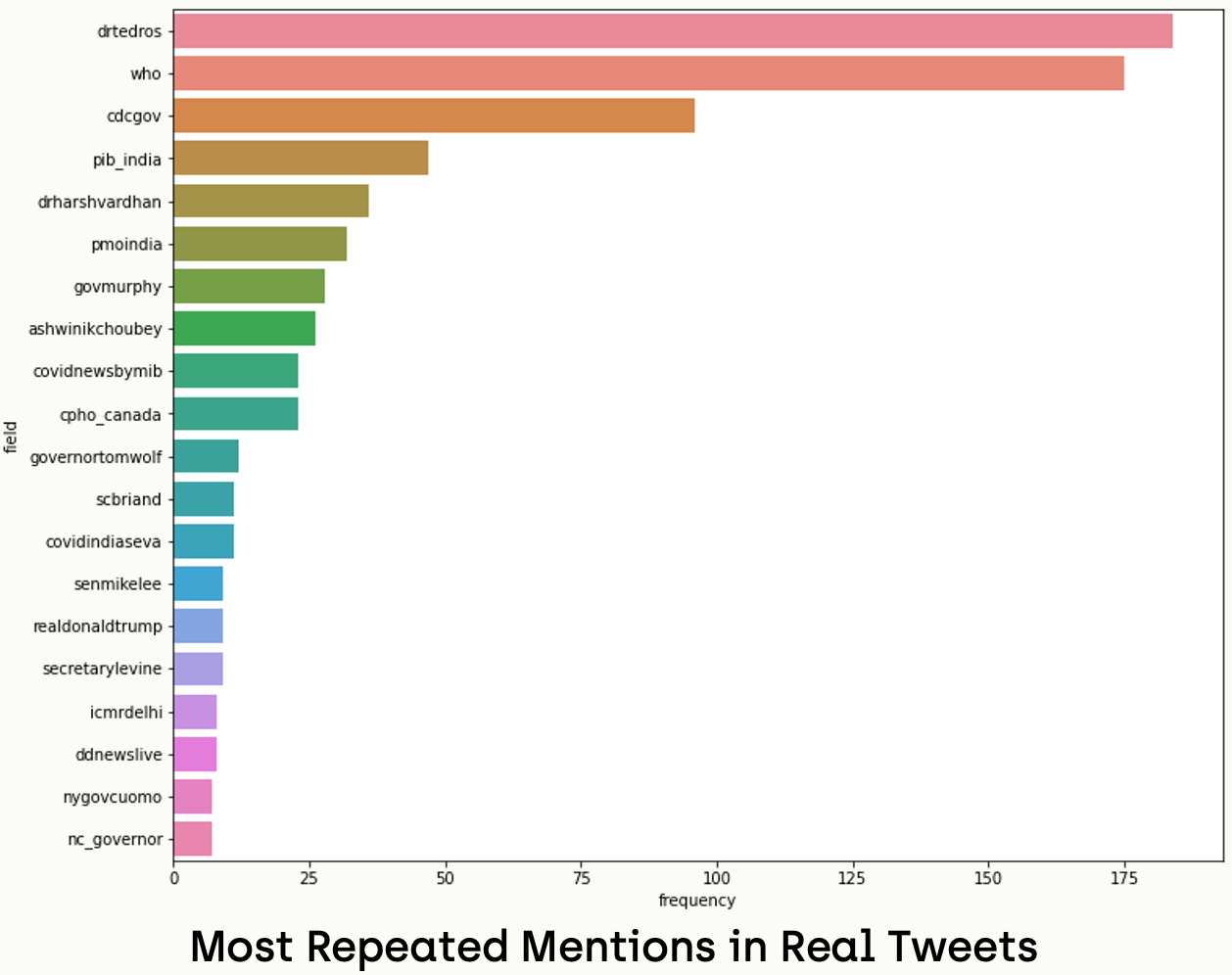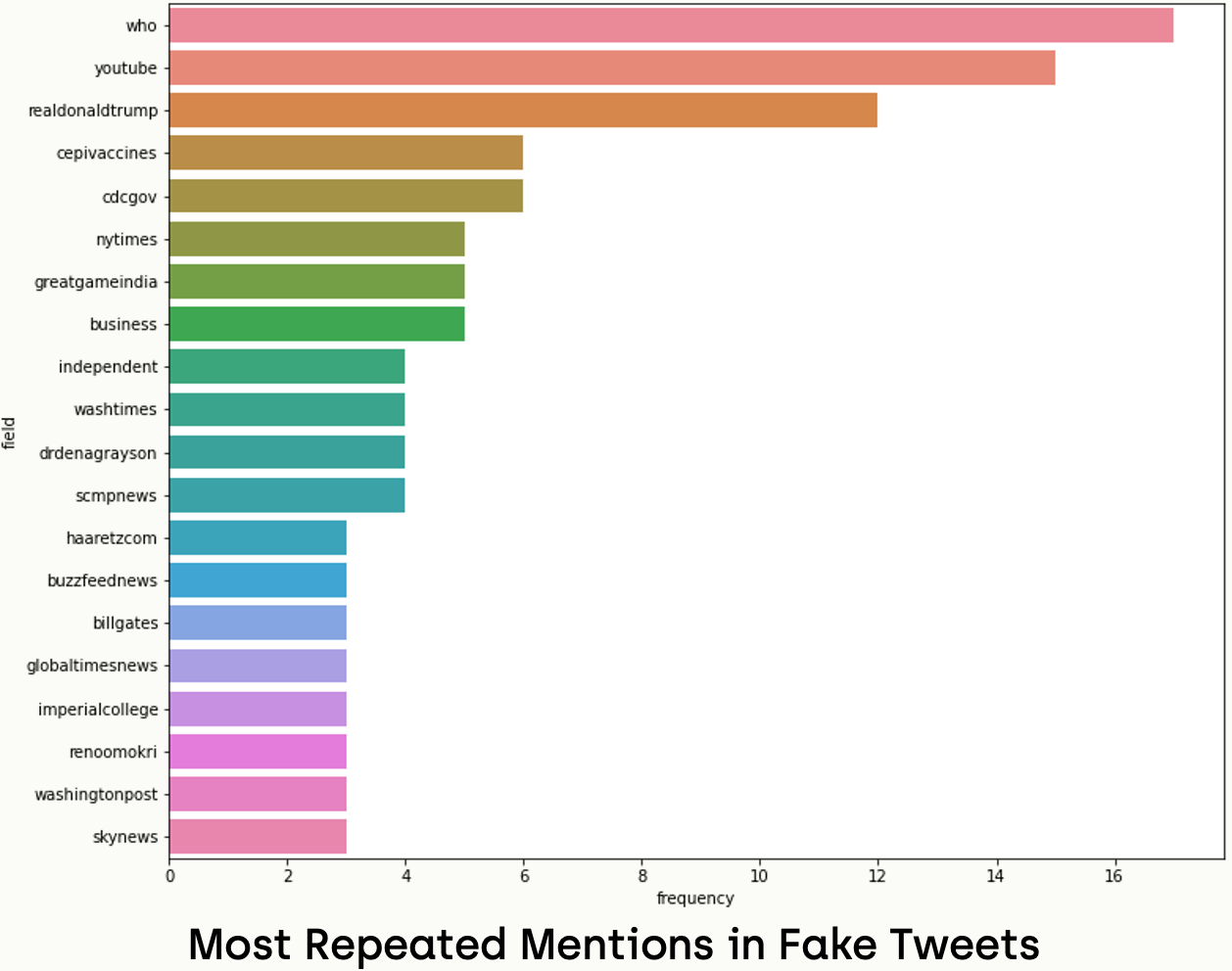We notice that the mentioned users in real news are reliable resources like Dr. Tedros; the Director-General of the World Health Organization, and CDC-Gov; the account of the Centers for Disease Control and Prevention. In comparison, the most mentioned accounts in fake news were unreliable resources, like youtube, realdonaldtrump.

### Step 11 - Numerical Fields Analysis

In this section, we will investigate the possible relations between the numerical values of real and fake news.

### Tweet Related Values

We can start by finding the average number of likes counts, reply count, and retweet count of each tweet in fake and real news datasets like the following:

``````fig4, ax4 = plt.subplots(nrows=1, ncols=3, figsize=(12, 7))

ax4.bar(["real", "fake"], [sub_real_tweets['like_count'].mean(),
sub_fake_tweets['like_count'].mean()], color=["aquamarine", "crimson"])
ax4.title.set_text("Average Like Count")

ax4.bar(["real", "fake"], [sub_real_tweets['retweet_count'].mean(
), sub_fake_tweets['retweet_count'].mean()], color=["aquamarine", "crimson"])
ax4.title.set_text("Average Retweet Count")

plt.show()``````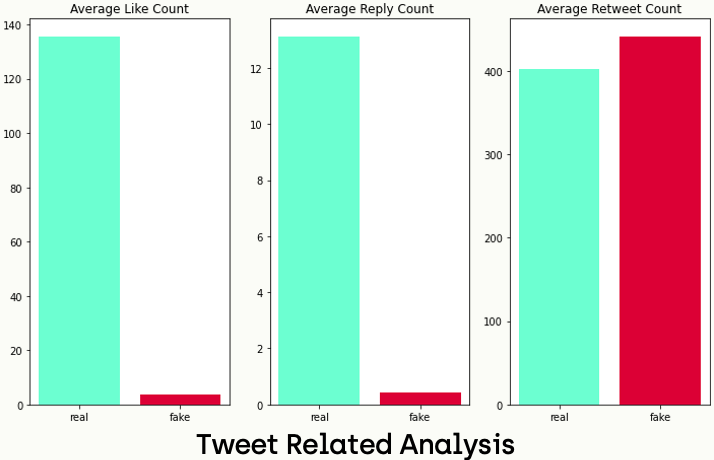According to the results above, we can say that real news tends to have a higher number of both like count and reply count, while fake news tends to have a higher number of retweets.

### User Related Values

On the other hand, we can do an analysis of the numbers that are related to the user, like follower counts, tweet counts, and following counts.

``````fig4, ax4 = plt.subplots(nrows=1, ncols=3, figsize=(12, 7))

ax4.bar(["real", "fake"], [sub_real_tweets['retweeter_followers_count'].mean(
), sub_fake_tweets['retweeter_followers_count'].mean()], color=["aquamarine", "crimson"])
ax4.title.set_text("Followers Count")

ax4.bar(["real", "fake"], [sub_real_tweets['retweeter_tweet_count'].mean(
), sub_fake_tweets['retweeter_tweet_count'].mean()], color=["aquamarine", "crimson"])
ax4.title.set_text("Tweet Count")

ax4.bar(["real", "fake"], [sub_real_tweets['retweeter_following_count'].mean(
), sub_fake_tweets['retweeter_following_count'].mean()], color=["aquamarine", "crimson"])
ax4.title.set_text("Following Count")

plt.show()``````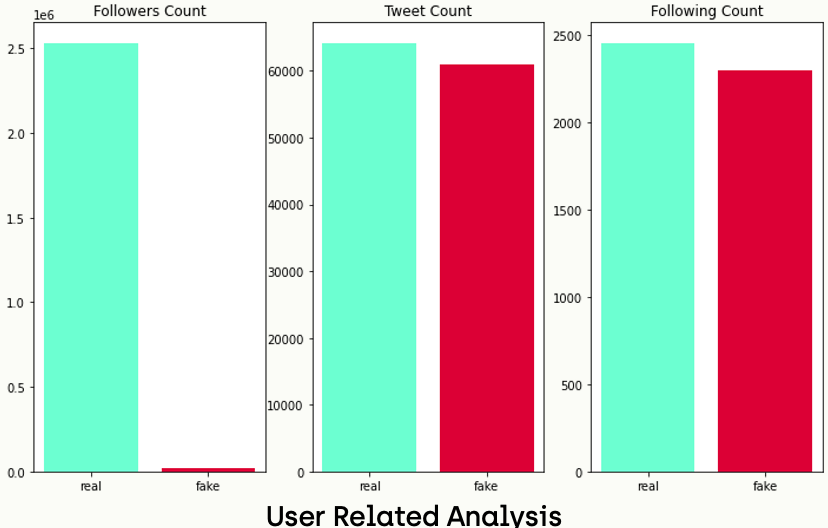Obviously, we can notice that accounts that are sharing real news have more followers than accounts that are sharing fake ones. On the other hand, there is no significant difference in the number of tweet counts and following counts between real and fake news publishers.

### Step 12 - Location Analysis

Since we did a lot of analysis of the numerical values, we can do geographical analysis depending on the location of the publisher accounts too. Let's start with the real news part.

``````location_freq_real = Counter(sub_real_tweets['location'])
location_freq_real_df = pd.DataFrame.from_records(location_freq_real.most_common(), columns=['location','frequency'])
fig, axes = plt.subplots(1,1,figsize=(12,10))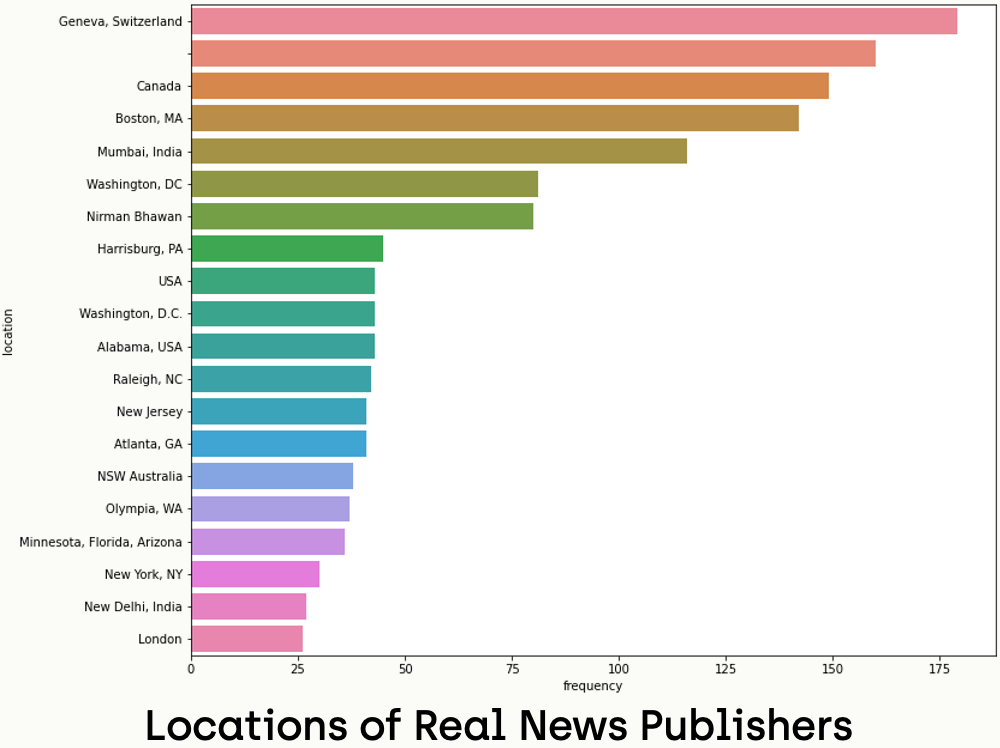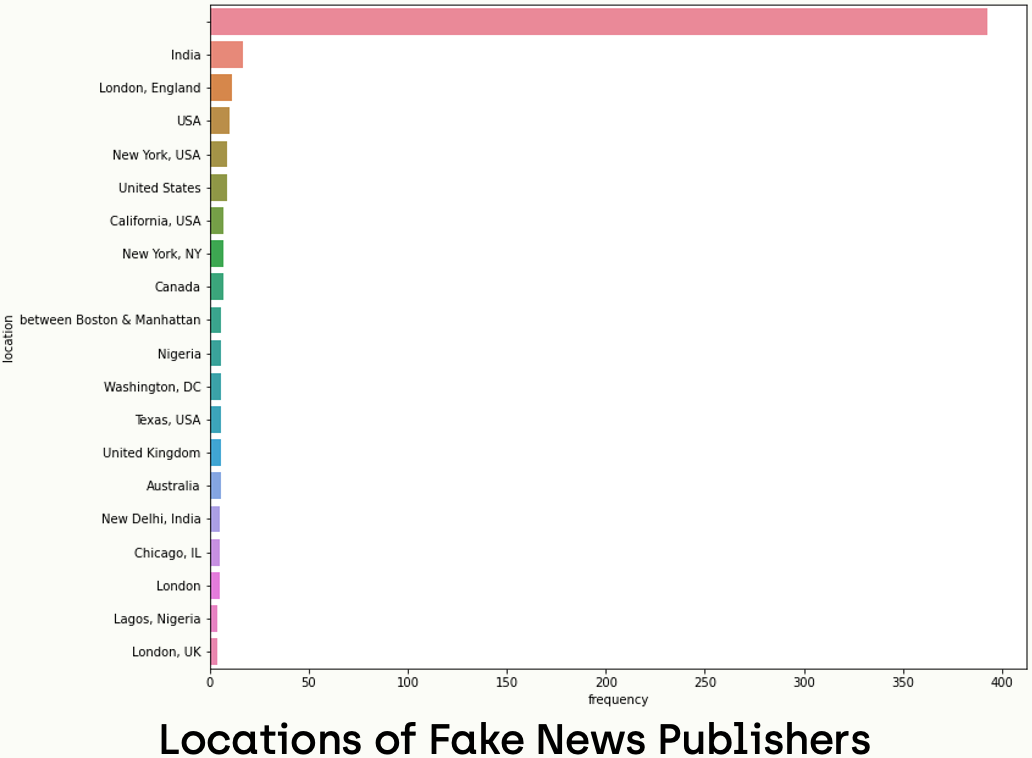In the case of real news, we can observe that accounts of most tweets show their locations, and only a small part of the data does not specify the location. In addition, the most repeated place is in Geneva, Switzerland, where the WHO (World Health Organization) shares its tweet. In the case of real news, we notice that a large percentage of the users have no information about the location. In addition, there is no clear dominance of the dataset.

### Step 13 - Owner Analysis

Last but not least, we will investigate the most repeated publisher among real and fake news by analyzing the owner_username field:

``````owner_username_freq_real = Counter(sub_real_tweets['owner_username'])
fig, axes = plt.subplots(1,1,figsize=(12,10))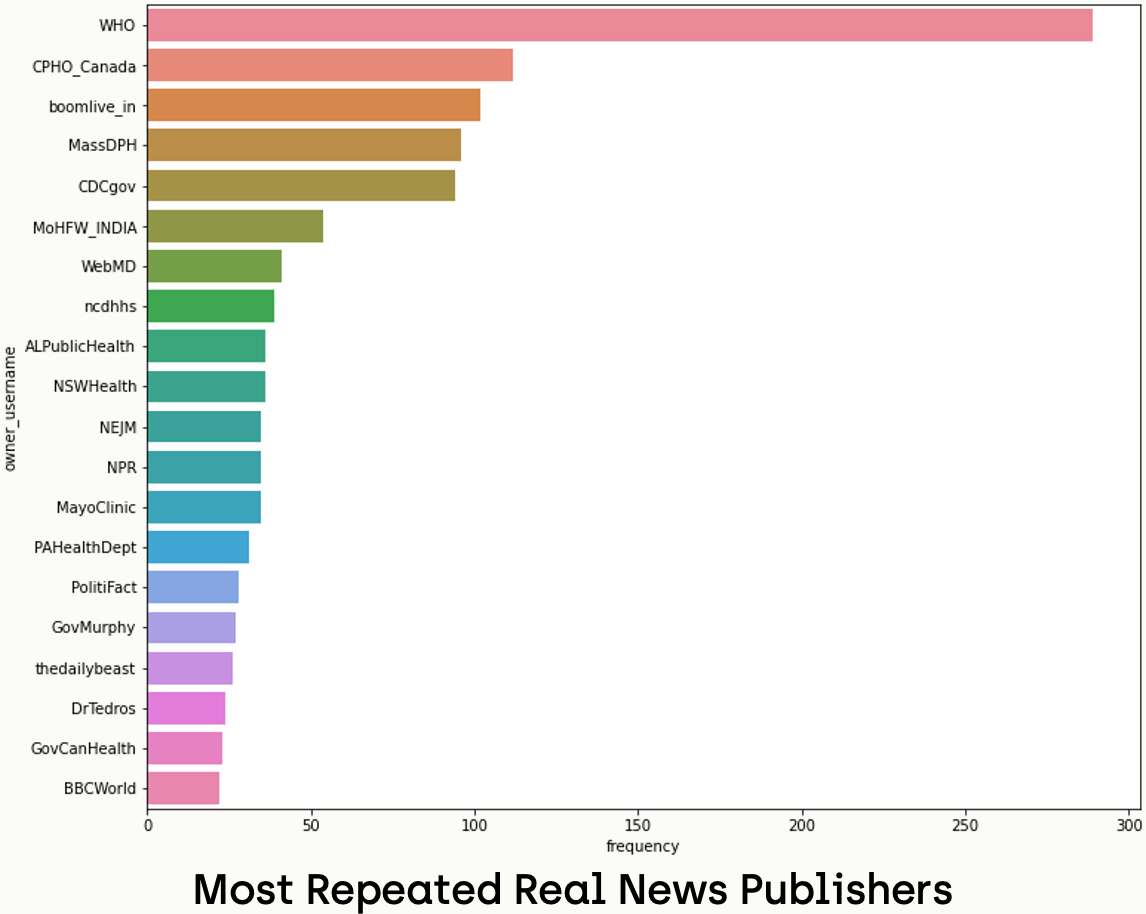By running the same script on fake news, we obtain the following chart: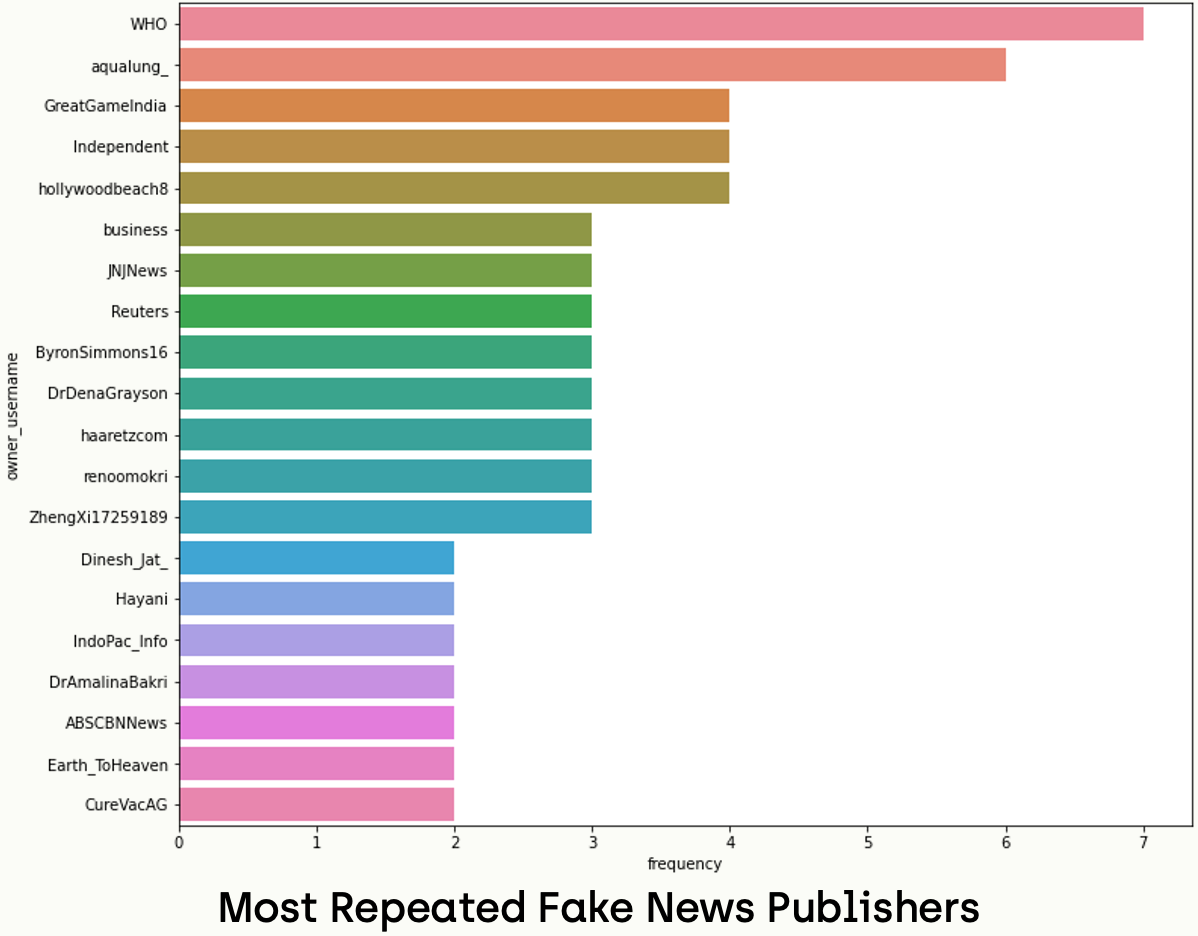We notice that the most repeated users in real news are reliable sources, and each account has a high number of tweets, so we can say that there is an obvious dominance in this case. On the other hand, we see that even the most repeated accounts do not have a high number of accounts, so we can say that there is no dominance in the case of fake news.

### Machine Learning-Based Model

In this section, we will train a machine learning-based model that takes the tweet's text as input and classifies it as real or fake news. Let's start by installing the required packages.

``````# in fnd environment
conda install -c anaconda scikit-learn
conda install -c anaconda scipy``````

### Data Preparation

Subsequently, we have to prepare the data for training by vectorizing them using the TF-IDF concept, which is a technique used to weight terms according to their importance of those terms within the document and corpus. Words that are frequent in a document but not across the corpus tend to have high TF-IDF scores.

``````from sklearn.feature_extraction.text import TfidfVectorizer
import numpy as np
import scipy.sparse as sp

tfidf_vectorizer = TfidfVectorizer(max_features=4000, ngram_range=(2,2))
X_real = tfidf_vectorizer.fit_transform(sub_real_tweets["text"])
X_fake = tfidf_vectorizer.fit_transform(sub_fake_tweets["text"])
X_combined = sp.vstack((X_real, X_fake))``````

Now, we have to prepare the output vector for training by creating a vector that contains the labels as 0 or 1;

``````y_real = np.repeat("real", X_real.shape)
y_fake = np.repeat("fake", X_fake.shape)
y_combined = np.concatenate([y_real,y_fake])
y_combined_binary = np.where(y_combined == "real", 1, 0)``````

As we have prepared the data for training, we can split it into training and validation sets. In this tutorial, we chose a 0.60/0.40 ratio for splitting the dataset. So, 60% of the data will be used for training and 40% for validation.

``````from sklearn.model_selection import train_test_split
X_train, X_test, y_train, y_test = train_test_split(X_combined, y_combined_binary, test_size=0.40, random_state=0)``````

Finally, we can initialize the model that we will train. The sklearn library provides too many machine learning models; for example, we will use DecisionTreeClassifier for this task.

### Training the Model

``````from sklearn.tree import DecisionTreeClassifier
decision_clf = DecisionTreeClassifier(random_state=42,min_samples_split=5, min_samples_leaf=1)
decision_clf.fit(X_train, y_train)
y_pred = decision_clf.predict(X_test)
print('Accuracy of Decision Tree classifier on test set: {:.2f}'.format(decision_clf.score(X_test, y_test)))``````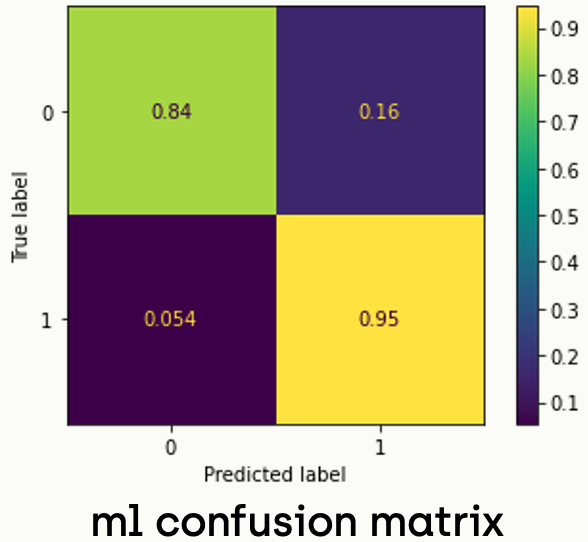### Deep Learning-Based Model

As we have seen in the previous section, using machine learning-based solutions is unsatisfactory. Therefore, we can train a deep learning-based network to get better classifiers with higher accuracy.

``````# in fnd environment
conda install -c anaconda tensorflow``````

### Data Preparation

Subsequently, we can start by representing our data as a one-hot representation, so every word (even symbols) which are part of the given text data is written in the form of vectors, constituting only 1 and 0. So one hot vector is a vector whose elements are only 1 and 0. Each word is written or encoded as a one-hot vector, with each one-hot vector being unique. This allows the word to be identified uniquely by its one-hot vector and vice versa. That is, no two words will have the same one-hot vector representation.

Required imports:

``````import tensorflow
import tensorflow.keras as keras
from tensorflow.keras.preprocessing.text import one_hot
import tensorflow.keras.layers as layers``````

Firstly, we have to combine the dataset, so we do the operations on one object.

``tweets_combined = list(sub_real_tweets["text"]) + list(sub_fake_tweets["text"])``

Subsequently, we prepare the one-hot representation vector and convert sequences to the same length, and then, we convert them to arrays and split them for the training phase:

``````vec_size = 5000
onehot_enc = [one_hot(words, vec_size)for words in tweets_combined]

max_length = 500

y_final=np.array(y_combined_binary)

X_train, X_test, y_train, y_test = train_test_split(X_final, y_final, test_size=0.40, random_state=42)``````

### Building The Network

Finally, since we have prepared the data, we can build our network. In this tutorial, we will build a simple network that consists of 4 layers; 1 embedding layer, 1 bidirectional LSTM layer, 1 dropout layer, and 1 dense layer. As hyperparameters, we will use a batch size of 64, Binary Crossentropy as a loss function, and Adam as an optimizer.

``````embedding_vector_features=40
model=tensorflow.keras.models.Sequential()

Add the following line to see the summary of the model:

``print(model.summary())``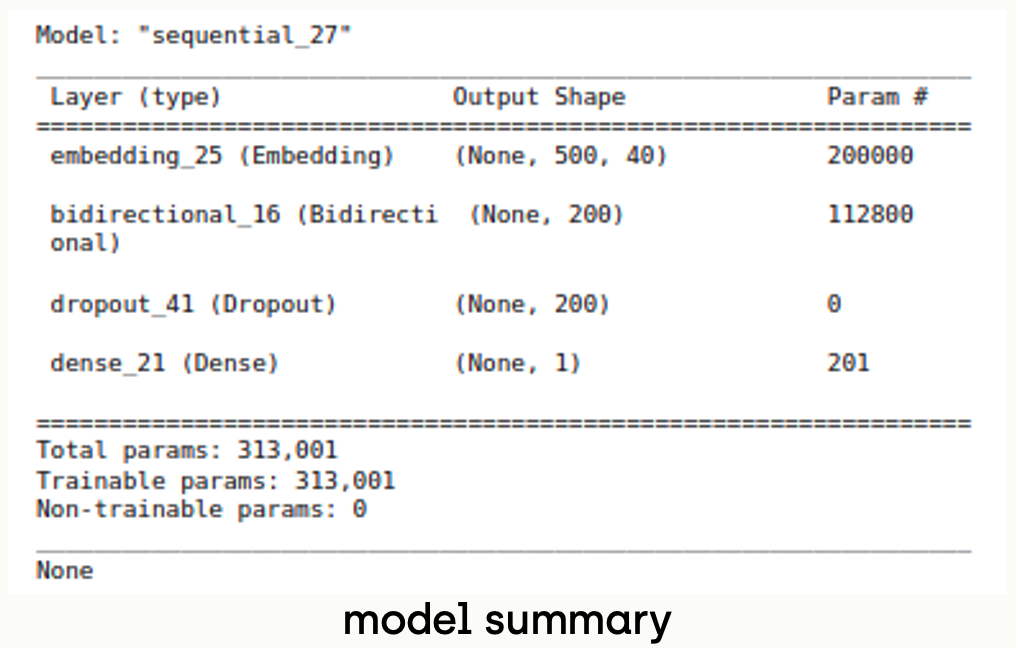### Training The Network

For stopping the training, we have 3 stopping criteria. The first one is the number of epochs; we will set it as 10; therefore, if we exceed the epoch threshold, the training is stopped. The second criterion is accuracy over the validation set; if it equals 95%, we stop the learning. The last one is the accuracy stability; if it gets stabilized for the subsequent 3 epochs without improving, we stop training. The best model is protected all over the training procedure. So even if the model gets bad by epochs, we can extract the best model, thanks to the EarlyStopping module of Keras.

``````class ThresholdCallback(keras.callbacks.Callback):
def __init__(self, threshold):
super(ThresholdCallback, self).__init__()
self.threshold = threshold

def on_epoch_end(self, epoch, logs=None):
accuracy = logs["val_accuracy"]
if accuracy >= self.threshold:
self.model.stop_training = True

callback=ThresholdCallback(threshold=0.95)
early_stop = tensorflow.keras.callbacks.EarlyStopping(monitor='accuracy', patience=3, restore_best_weights=True)
history = model.fit(X_train, y_train, validation_data=(X_test,y_test), epochs=10, batch_size=64, verbose=1, callbacks=[callback, early_stop])``````

### Evaluation

To evaluate the model, we have to run the model against the validation set and plot the confusion matrix.

``````from sklearn import metrics

def plot_matrix(cm, classes, title):
ax = sns.heatmap(cm, cmap="Blues", annot=True, xticklabels=classes, yticklabels=classes, cbar=False)
ax.set(title=title, xlabel="predicted label", ylabel="true label")

predictions = (model.predict(X_test) > 0.5).astype("int32")
cm = metrics.confusion_matrix(y_test, predictions)

cm_norm = cm / cm.astype(np.float).sum(axis=1)
plot_matrix(cm_norm, ["0", "1"],"cm" )``````

Running the code above gives a confusion matrix like the following: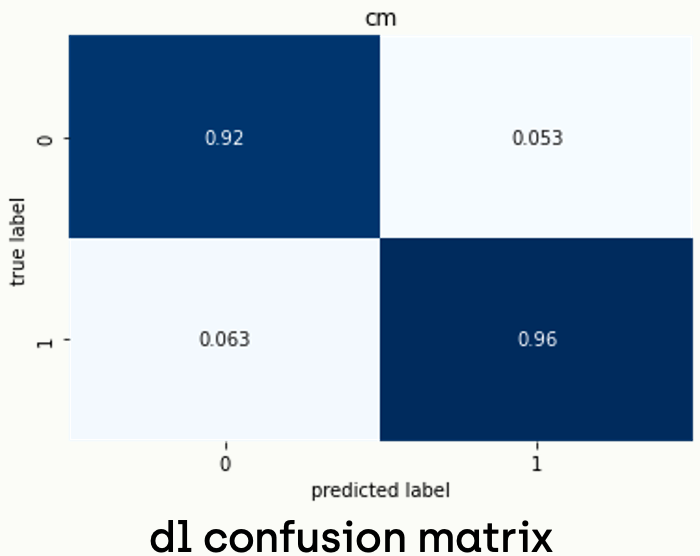Finally! We trained a deep learning-based model and got a validation accuracy of 94%. Now, we can save the model from keeping the trained weights.

``model.save("fnd_best.h5")``
##### Notes

-       It is not mandatory to use this network architecture and play with the layers and parameters to get better accuracy or faster models.

-       Running the code above may not give exactly the same accuracy because the initial weights are randomly assigned.

## Conclusion

After carrying out this tutorial, we can conclude that even if we have limited details about tweets in our dataset, we can get more fields to enrich the dataset in our projects using Twitter API. Also, in our analysis, we noticed that fetching new details about tweets can give us further insights into the data. So, we can discover the possible relations between fields and interpret them in the context of the research field.

As already pointed out in the model's part, we found that it is possible to create a model with an acceptable performance that can automatically distinguish fake news using machine learning-based methods. Besides, we can see that the deep learning-based model provides a more stable and more satisfactory performance in the subject that we investigated. All codes are included in a notebook in the GitHub repository.### Get Started

Get started! Build better data, now.